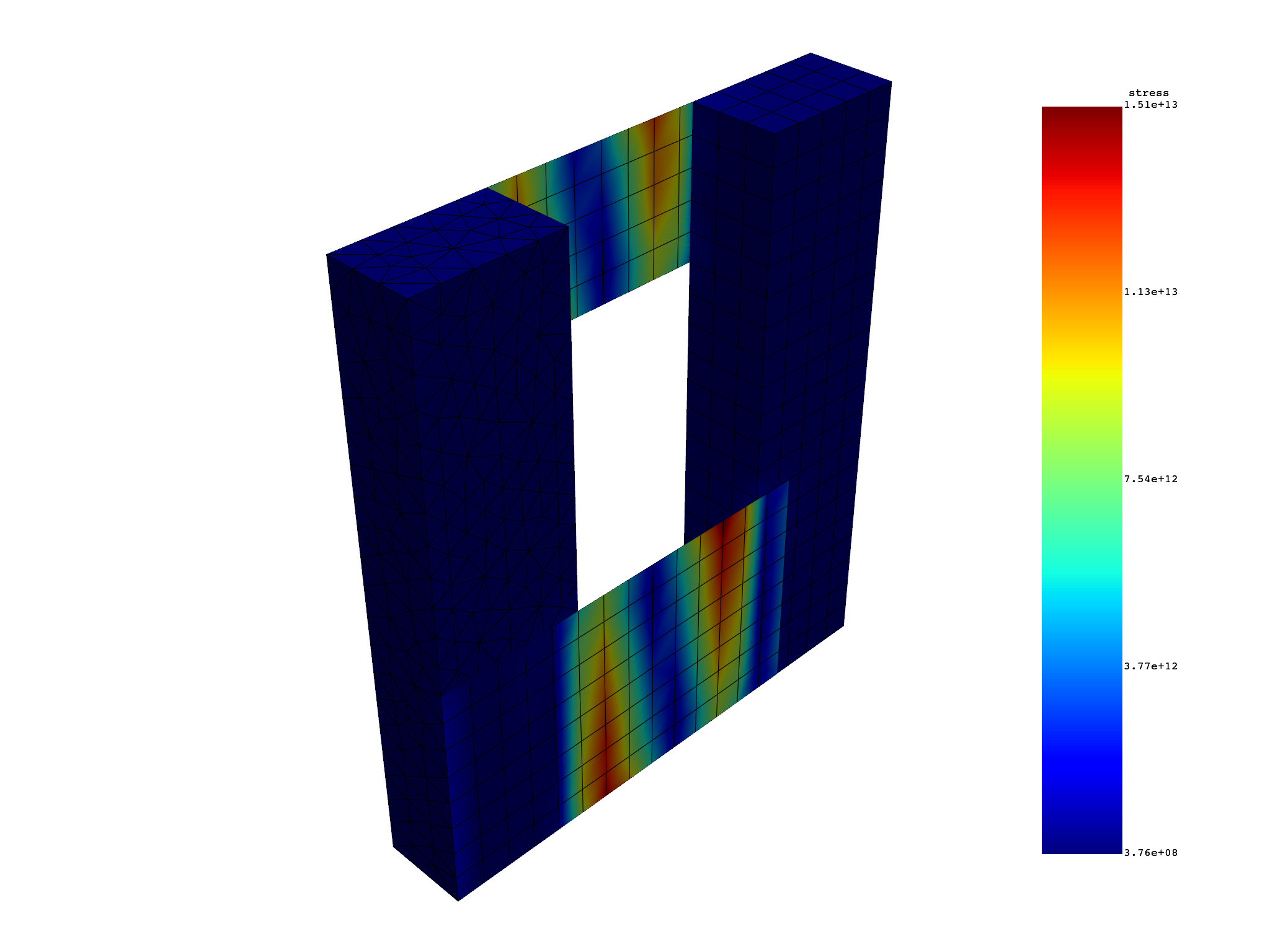# PyDPF-Post overview#

This example provides an overview of how you use PyDPF-Post.

## Perform required imports#

Perform required imports. # This example uses a supplied file that you can get by importing the DPF `examples` package.

```from ansys.dpf import post
from ansys.dpf.post import examples
```

## Get `Solution` object#

Get the `Solution` object that allows access to the result. The `Solution` object must be instantiated with the path for the result file. For example, `"C:/Users/user/my_result.rst"` on Windows or `"/home/user/my_result.rst"` on Linux.

```solution = post.load_solution(examples.multishells_rst)
```

## Get mesh and time frequency support#

Get the mesh and time frequency support. The mesh is the support of the model. The time frequency support is the time/frequency representation of the model.

```mesh = solution.mesh
time_freq_support = solution.time_freq_support
```

## Get `Result` object#

Get a `Result` object from the `Solution` object. The `Result` object can be a stress, plastic strain, elastic strain, temperature, or displacement.

```post.print_available_keywords()
stress = solution.stress(location=post.locations.nodal, time_scoping=)

# Both location and ``time_scoping`` are available in the definition of
# the stress result.

stress.definition.location
stress.definition.time_scoping

print(stress)
```
```element_scoping: list, int or dpf.core.Scoping
grouping: str. Use post.grouping.(...) as helper.
location: str. Use post.locations.(...) as helper.
mapdl_grouping: int. Write 186 to get mapdl_elements solid_186.
named_selection: str. Name of named_selection.
node_scoping: list, int or dpf.core.Scoping
path: DpfPath object that
contains a list of coordinates,
e.g. [[0.1, 0.0, 0.0],
[0.0, 0.1, 0.0]].
set: int
time: float
time_scoping: list, int or dpf.core.Scoping

Stress Tensor object.

Object properties:
- location   : Nodal
- time_scoping : 
```

## Compute data#

Compute data.

SX subresult

This code gets the subresult `SX`, which is the stress tensor in the XX direction.

```sx = stress.xx
sx.num_fields
sx_field = sx
sx_data = sx.get_data_at_field(0)
print("Length of the data:", len(sx_data))
print()
print("Maximum Stress Field:\n", sx.max)
print()
print("Maximum data at stress field:", sx.max_data)
print("Maximum SX at Field 0:", sx.get_max_data_at_field(0))
```
```Length of the data: 6854

Maximum Stress Field:
DPF stress_1.s0 Field
Location: Nodal
Unit: Pa
2 entities
Data: 1 components and 2 elementary data

IDs                   data(Pa)
------------          ----------
0                     6.676153e+11

1                     1.536714e+13

Maximum data at stress field: [6.67615284e+11 1.53671424e+13]
Maximum SX at Field 0: 667615283882.6666
```

Stress tensor result

This code gets the minimum and maximum stresses at a field for all directions (`XX`, `XY`, `XZ`, `XY`, `YZ`, and `XZ`.

```s = stress.tensor
s_field = s
s_data = sx.get_data_at_field(0)
print("Length of the data:", len(s_data))
print()
print("Maximum stress field:\n", s.max)
print()
print("Maximum data at stress field:", s.max_data)
print("Maximum stress tensors at field 0:\n", s.get_max_data_at_field(0))

print("Minimum stress field:\n", s.min)
print()
print("Minimum data at stress field:", s.min_data)
print("Minimum stress tensors at field 0:\n", s.get_min_data_at_field(0))
```
```Length of the data: 6854

Maximum stress field:
DPF stress_1.s Field
Location: Nodal
Unit: Pa
2 entities
Data: 6 components and 2 elementary data

IDs                   data(Pa)
------------          ----------
0                     6.676153e+11   8.744609e+11   4.953944e+11   6.018891e+11   1.103258e+11   1.855802e+11

1                     1.536714e+13   4.217815e+08   3.782022e+12   2.079505e+11   1.579960e+11   1.099721e+12

Maximum data at stress field: [[6.67615284e+11 8.74460905e+11 4.95394439e+11 6.01889079e+11
1.10325826e+11 1.85580244e+11]
[1.53671424e+13 4.21781549e+08 3.78202198e+12 2.07950475e+11
1.57995981e+11 1.09972131e+12]]
Maximum stress tensors at field 0:
[6.67615284e+11 8.74460905e+11 4.95394439e+11 6.01889079e+11
1.10325826e+11 1.85580244e+11]
Minimum stress field:
DPF stress_1.s Field
Location: Nodal
Unit: Pa
2 entities
Data: 6 components and 2 elementary data

IDs                   data(Pa)
------------          ----------
0                     -5.779078e+11  -7.356049e+11  -2.412009e+11  -2.981459e+11  -2.421431e+11  -3.091386e+11

1                     -1.496015e+13  -2.636956e+08  -3.779456e+12  -3.354327e+11  -1.571902e+11  -9.661232e+11

Minimum data at stress field: [[-5.77907764e+11 -7.35604867e+11 -2.41200931e+11 -2.98145939e+11
-2.42143056e+11 -3.09138563e+11]
[-1.49601458e+13 -2.63695614e+08 -3.77945626e+12 -3.35432675e+11
-1.57190152e+11 -9.66123233e+11]]
Minimum stress tensors at field 0:
[-5.77907764e+11 -7.35604867e+11 -2.41200931e+11 -2.98145939e+11
-2.42143056e+11 -3.09138563e+11]
```

## Plot result#

Plot a result by using the `plot_contour()` method.

```s.plot_contour()
```Total running time of the script: ( 0 minutes 0.759 seconds)

Gallery generated by Sphinx-Gallery